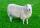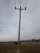# Trip

On the trip drank 3/10 of pupils tea, 2/5 cola, 1/4 mineral water and remaining 3 juice. How many students were on the trip?

Result

n =  60

#### Solution:

3/10 n + 2/5 n + 1/4 n + 3 = n

n = 60

n = 60

Calculated by our simple equation calculator.

Leave us a comment of example and its solution (i.e. if it is still somewhat unclear...):

Showing 0 comments:Be the first to comment!#### To solve this example are needed these knowledge from mathematics:

Need help calculate sum, simplify or multiply fractions? Try our fraction calculator. Do you have a linear equation or system of equations and looking for its solution? Or do you have quadratic equation?

## Next similar examples:

1. Cleaning windowsCleaning company has to wash all the windows of the school. The first day washes one-sixth of the windows of the school, the next day three more windows than the first day and the remaining 18 windows washes on the third day. Calculate how many windows ha
2. PearsThere were pears in the basket, I took two-fifths of them, and left six in the basket. How many pears did I take?
3. FactoryIn the factory workers work in three shifts. In the first inning operates half of all employees in the second inning and a third in the third inning 200 employees. How many employees work at the factory?
4. PipeSteel pipe has a length 2.5 meters. About how many decimetres is 1/3 less than 4/8 of this steel pipe?
5. The farmerThe farmer had 140 sheep. For the next year, she decided to change the number of sheep in ratio 10: 7. How many sheep will he have then?
6. EquationSolve the equation: 1/2-2/8 = 1/10; Write the result as a decimal number.
7. FractionsThree-quarters of an unknown number are 4/5. What is 5/6 of this unknown number?
8. Unknown numberI think the number - its sixth is 3 smaller than its third.
9. Simple equationSolve for x: 3(x + 2) = x - 18
10. Equation with fractionsSolve equation: ? It is equation with fractions.
11. UN 1If we add to an unknown number his quarter, we get 210. Identify unknown number.
12. EqnSolve equation with fractions: 2x/3-50=40+x/4
13. Equation with xSolve the following equation: 2x- (8x + 1) - (x + 2) / 5 = 9
14. Simple equation 6Solve equation with one variable: X/2+X/3+X/4=X+4
15. Unknown numberIdentify unknown number which 1/5 is 40 greater than one tenth of that number.
16. Iron poleThe iron pole is in the ground 2/5 of its length, partly above the ground 1/3 is yellow, and the unpainted section is 6 m long. How long is the entire column?
17. TogetherGrandfather, father, and son are carpenters. They decided to make a wardrobe. Father produces it for 12 hours, his son for 15 hours, and grandfather for 10 hours. How long will they make it together?